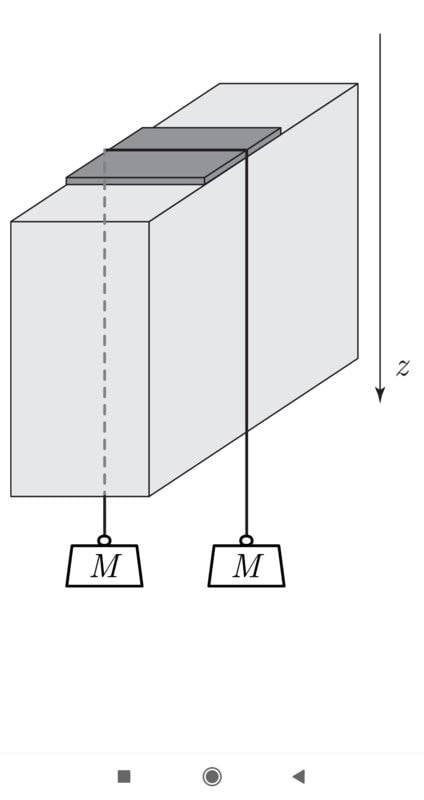# Entropy change when melting ice then refreezing the water

yamata1
Homework Statement:
A bloc of ice at temperature 273 K and height h has a plate of surface S with symetrically distributed weights of mass M hanging on both sides, so constant pressure is applied to the bloc and begins melting,the water rises from under the plate and refreezes above the plate.
What is the entropy change ##dS_1## of a mass dm of ice melting?
What is the entropy change ##dS_2## of a mass dm of water refreezing?
In using the fact that ##|\Delta T |<< T_0##, deduce therefrom the rate of change of total entropy; what is its sign (comment)?
Show that the total variation of entropy during the complete process where the plate has passed through the ice is independent of ##L_f## and is worth ##∆S=\frac{2Mgh(v_l-v_s)}{V_g T_0}##
Let ##P1 = P_0 + ∆P## and ##T_1 = T_0 + ∆T## be the equilibrium pressure and temperature of the ice under the plate. We denote by ##L_f## the specific latent heat of fusion of the ice, ##v_l## and ##v_s ## the volumes mass of liquid water and ice, g acceleration of gravity. We will assume that variations in temperature and equilibrium pressure on either side of the plate are weak enough that ##L_f##,##v_l## and ##v_s## can be considered constant, their values being those of the state (##T_0, P_0##).
Relevant Equations:
##L_f=\frac{Q}{m} \; \; ##,##∆T=\frac{T(v_l-v_s)∆P}
{L}## I dont know how to express the total entropy change.

Thank you for help
##dmL_f= Q \; \; ##,##∆T=\frac{T(v_l-v_s)∆P}{L} \; \;##,##\frac{dmL_f}{T_0}= dS_2 \; \;##,##\frac{dmL_f}{T_1}= dS_1 ##

Mentor
Can you please provide a diagram.

Homework Helper
Gold Member
Can you please provide a diagram.
I agree. A diagram would help.

Also, @yamata1, could you explain what $V_g$ is? It's one of the variables in your change of entropy equation, but I don't see a definition for it. Is it the absolute volume of the block of ice (when there's no water)?

[Edit: Or maybe $V_g$ is the absolute volume of the path that the plate eventually moves through, if the plate has a smaller area than the block's area. That's just a guess. Can you confirm? (In other words, is $V_g = hS$, where $S$ here is the surface area of the plate, not entropy?)]

Last edited:
yamata1Can you please provide a diagram.

yamata1
I agree. A diagram would help.

Also, @yamata1, could you explain what $V_g$ is? It's one of the variables in your change of entropy equation, but I don't see a definition for it. Is it the absolute volume of the block of ice (when there's no water)?

[Edit: Or maybe $V_g$ is the absolute volume of the path that the plate eventually moves through, if the plate has a smaller area than the block's area. That's just a guess. Can you confirm? (In other words, is $V_g = hS$, where $S$ here is the surface area of the plate, not entropy?)]
It's a mistake, it's supposed to be ##v_s##, the ##L##'s are ##L_f##'s also.

Last edited:
Homework Helper
Gold Member
Homework Equations:: ##L_f=\frac{Q}{m} \; \; ##,##∆T=\frac{T(v_l-v_s)∆P}
{L}## I don't know how to express the total entropy change.

Thank you for help

##dmL_f= Q \; \; ##,##∆T=\frac{T(v_l-v_s)∆P}{L} \; \;##,##\frac{dmL_f}{T_0}= dS_2 \; \;##,##\frac{dmL_f}{T_1}= dS_1 ##
Looks like you have all the reltionships that you need. You say that you don't know how to express the total entropy change. Doesn't ##dS_{\rm tot} = dS_1 + dS_2##? Think about the signs of ##dS_1## and ##dS_2##. Which one of these is a negative quantity? You will need to make an approximation for ##dS_1## based on the fact that ##|\Delta T| \ll T_0##.

Last edited:
•yamata1
yamata1
Looks like you have all the reltionships that you need. You say that you don't know how to express the total entropy change. Doesn't ##dS_{\rm tot} = dS_1 + dS_2##? Think about the signs of ##dS_1## and ##dS_2##. Which one of these is a negative quantity? You will need to make an approximation for ##dS_1## based on the fact that ##\Delta T \ll T_0##.
##dS_2+dS_1=-\frac{dmL_f} {T_0}+\frac{dmL_f} {T_0+∆T}=-\frac{dmL_f} {T_0}+\frac{dmL_f} {T_0}(1-\frac{∆T} {T_0})=
-dmL_f\frac{∆T}{{T_0}^2}=-\frac{dm(v_l-v_s)∆P} {T_0}=\frac{dm(v_s-v_l)2Mg} {ST_0}=\frac{(v_s-v_l)2Mgh} {v_s T_0} =∆S\; \; \;## since ##v_s=\frac{Sh}{dm}## I made another mistake while typing ##∆S## in my first post, it was supposed to be ##∆S=\frac{(v_s-v_l)2Mgh} {v_s T_0}##

Homework Helper
Gold Member
##dS_2+dS_1=-\frac{dmL_f} {T_0}+\frac{dmL_f} {T_0+∆T}=-\frac{dmL_f} {T_0}+\frac{dmL_f} {T_0}(1-\frac{∆T} {T_0})=
-dmL_f\frac{∆T}{{T_0}^2}=-\frac{dm(v_l-v_s)∆P} {T_0}=\frac{dm(v_s-v_l)2Mg} {ST_0}=\frac{(v_s-v_l)2Mgh} {v_s T_0} =∆S\; \; \;## since ##v_s=\frac{Sh}{dm}## I made another mistake while typing ##∆S## in my first post, it was supposed to be ##∆S=\frac{(v_s-v_l)2Mgh} {v_s T_0}##
I think that looks pretty good. I interpreted ##dS_1## and ##dS_2## to be infinitesimal entropy changes due to the melting and refreezing of an infinitesimal mass ##dm##. Then, you have shown that

##dS_{\rm tot} = dS_2+dS_1=\frac{dm(v_s-v_l)2Mg} {ST_0}##

To get the finite change ##\Delta S_{\rm tot}## for the entire process, you can integrate: ##\Delta S_{\rm tot} = \int dS_{\rm tot}##

This will yield the same result that you got if you use ##v_s=\frac{Sh}{m_{\rm ice}}##, where ##m_{\rm ice}## is the mass of the total amount of ice that melted and refroze during the process.

yamata1
I think that looks pretty good. I interpreted ##dS_1## and ##dS_2## to be infinitesimal entropy changes due to the melting and refreezing of an infinitesimal mass ##dm##. Then, you have shown that

##dS_{\rm tot} = dS_2+dS_1=\frac{dm(v_s-v_l)2Mg} {ST_0}##

To get the finite change ##\Delta S_{\rm tot}## for the entire process, you can integrate: ##\Delta S_{\rm tot} = \int dS_{\rm tot}##

This will yield the same result that you got if you use ##v_s=\frac{Sh}{m_{\rm ice}}##, where ##m_{\rm ice}## is the mass of the total amount of ice that melted and refroze during the process.
Yes, so we do have a gain in entropy.Thanks again.